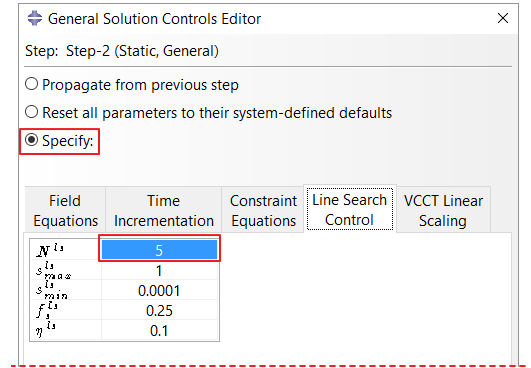## Blog

### Some tips for solving nonlinear problems in Abaqus

Obtaining a solution for nonlinear problems is always a challenge in any FEA software. It is very common to encounter convergence difficulties. Here are some tips to overcome such difficulties for the user of Abaqus.

### 1. Simplified model

Start with a simplified model and build it by adding details gradually, e.g. plasticity and friction can be excluded in the beginning. As the simplified model works well, details can be added one-by-one. It makes easier to find out a source of convergence difficulty and hence fix it.

### 2.  Displacement Control

In many cases, not all the degrees of freedom of contacting parts are constrained  expecting that contact will prevent unconstrained rigid body motion. For such problems it is recommended to use displacement control instead of load control to establish the initial contact. For a load control contact problem with initial contact not established, unconstrained rigid body motions may occur. An alternative is to introduce viscous damping at the contact interface to eliminate the rigid body motion.

### 3.  Increment size

For highly nonlinear problems set the initial increment size sufficiently small. Also set the maximum increment size to a small value in case a sudden stiffness change is expected otherwise it can jump across critical points and may need more iterations to converge.

### 4.  Plasticity

Abaqus assumes perfectly plastic behavior after the last specified data point of the stress-strain curve. Once a region reaches that point, it cannot resist further deformation as it has no stiffness. It could cause convergence difficulties. It is suggested to add an additional data point with a large strain such that last curve has slightly increasing slope.

### 5.  Incompressible material

Use hybrid elements for nearly incompressible material.

### 6.Unsymmetric solver

If the friction is included in a model, it adds unsymmetric terms to the system of equations. By default, if the coefficient of friction is less than 0.2, Abaqus/Standard uses the symmetric solver and if coefficient of friction is higher than 0.2, unsymmetric solver is invoked. It is assumed that when the coefficient of friction is low, unsymmetric terms are quite small and symmetric solver works well. For higher coefficients of friction, unsymmetric terms becomes significant and the unsymmetric solver can improve the convergence rate.

For problems with relative finite sliding of contacting surfaces using “surface-to-surface” discretization, unsymmetric terms could become significant even when coefficient of friction is less than 0.2, resulting in slower or even no convergence. In such cases, it is imperative to invoke the unsymmetric solver to overcome convergence difficulties.Figure 1: Invoking unsymmetric solver

In general, unsymmetric solver is more expensive than symmetric solver for each iteration, however, often there are fewer iterations required with the unsymmetric solver and achieves faster rate of convergence.

### 7.  Volume-proportional damping

For locally unstable quasi-static problems, use automatic stabilization capability to dissipate the strain energy. Automatic stabilization applies volume-proportional damping to stabilize the model.Figure 2: Specifying automatic stabilization for problems with local instabilities

### 8.  Line search

In strongly nonlinear problems the Full Newton solution technique, which is used in Abaqus/Standard by default, may sometimes diverge during equilibrium iterations. To handle such difficulties, Abaqus provides the line search algorithm which could be considered as convergence enhancement technique. The line search algorithm detects divergence and applies a scale factor to the computed displacement correction. The aim is to find a better configuration which would help to overcome divergence.

By default, the line search algorithm is not enabled when using Full Newton method. The  line search procedure can be activated by setting  parameter to a reasonable value as shown in the figure below. Here represents the maximum number of line search iterations.Figure 3: Activating line search algorithm

Line search is not only useful in situations where equilibrium is not achieved due to divergence but it can also increase the convergence rate for the problems with slow convergence.

If you want to learn Abaqus in depth, you could have a look at the online course “Solving Non-linear Problems with Abaqus